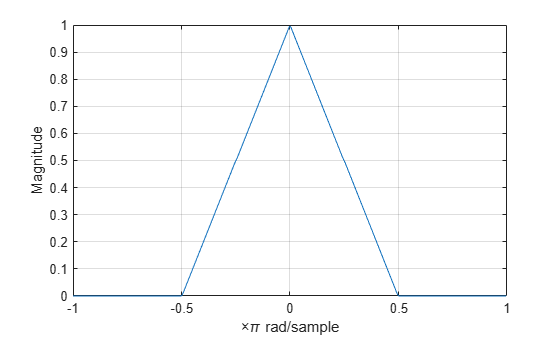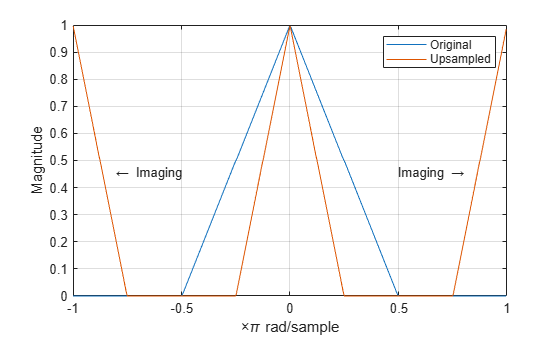Documentation

## Upsampling — Imaging Artifacts

This example shows how to upsample a signal and how upsampling can result in images. Upsampling a signal contracts the spectrum. For example, upsampling a signal by 2 results in a contraction of the spectrum by a factor of 2. Because the spectrum of a discrete-time signal is $2\pi$-periodic, contraction can cause replicas of the spectrum normally outside of the baseband to appear inside the interval $\left[-\pi ,\pi \right]$.

Create a discrete-time signal whose baseband spectral support is $\left[-\pi ,\pi \right]$. Plot the magnitude spectrum.

F = [0 0.250 0.500 0.7500 1]; A = [1.0000 0.5000 0 0 0]; Order = 511; B = fir2(Order,F,A); [Hx,W] = freqz(B,1,8192,'whole'); Hx = [Hx(4098:end) ; Hx(1:4097)]; omega = -pi+(2*pi/8192):(2*pi)/8192:pi; plot(omega,abs(Hx))Upsample the signal by 2. Plot the spectrum of the upsampled signal.

y = upsample(B,2); [Hy,W] = freqz(y,1,8192,'whole'); Hy = [Hy(4098:end) ; Hy(1:4097)]; hold on plot(omega,abs(Hy),'r','linewidth',2) xlim([-pi pi]) legend('Original Signal','Upsampled Signal') xlabel('Radians/Sample') ylabel('Magnitude') text(-2,0.5,'\leftarrow Imaging','HorizontalAlignment','center') text(2,0.5,'Imaging \rightarrow','HorizontalAlignment','center') hold offYou can see in the preceding figure that the contraction of the spectrum has drawn subsequent periods of the spectrum into the interval $\left[-\pi ,\pi \right]$.